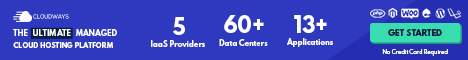# Growth Rates

## Small Differences in Economic Growth Rates are Important

The difference between 1.3 percent and 2.3 percent may seem trivial but, over long periods, small differences in growth rates can have a large impact.

For example, suppose you have \$100 in a savings account earning an annual interest rate of 1.3 percent, which means you will receive an interest payment of \$1.30 this year. If the interest rate Opens in new window on the account is 2.3 percent you will earn \$2.30.

The difference of an extra \$1.00 interest payment seems significant.

But if you leave the interest as well as the original \$100 in your account for another year, the difference becomes greater because now the higher interest is applied to a larger amount—\$102.30—and the lower interest rate is applied to a smaller amount—\$101.30.

This process, known as compounding, magnifies even small differences in interest rates over long periods of time.

Over a period of 50 years, your \$100 would grow to \$312 at an interest rate of 2.3 percent but to only \$191 at an interest rate of 1.3 percent.

The principle of compounding applies to economic growth rates Opens in new window as well as interest rates.

For example, in 1950 real GDP per capita in Argentina was \$5474 (measured in US 2005 dollars), which was larger than Italy’s real GDP per capita of US\$5361. Over the next 60 years the economic growth rate in Italy averaged 2.8 percent per year, while in Argentina the growth rate was only 1.4 percent per year.

Although this difference in growth rates of only 1.4 percentage points may seem small, in 2010 real GDP per capita in Italy had risen to US\$27930, while real GDP per capita in Argentina was only US\$12931.

In other words, because of a relatively small difference in the growth rates of the two economies the standard of living of the typical person in Italy went from being below that of the typical person in Argentina to being much higher.

The important point to keep in mind is this:

In the long run, small differences in economic growth rates result in big differences in living standards.Don’t confuse average annual percentage change with total percentage change

When economists talk about growth rates over a period of more than one year, the numbers are always average annual percentage changes and not total percentage changes.

For example, real GDP in Australia was approximately \$236 billion in 1960 and \$1493 billion in 2013. The percentage change in real GDP between these two years is:Average 533 percent over 53 years would give an incorrect measure of the annual growth rate of 10.1 percent. However, this is not the growth rate between the two years.

The growth rate between these two years is the rate at which \$236 billion in 1960 would have to grow on average each year to end up as \$1493 billion in 2013, which is 5.54 percent [calculated by using a compounding calculator].